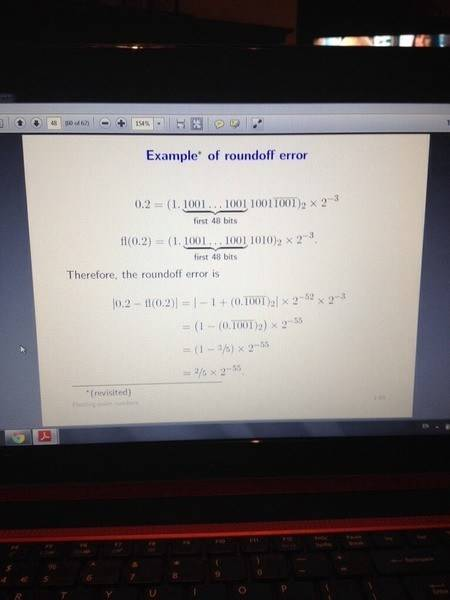# Round off error of floating point number.

• SherlockOhms

#### SherlockOhms

(Mods, I posted a similar thread in the computer science forum but now realize that this is a more suitable place for it. Could you please remove said thread from the other forum)

I've attached a photo below of the example. 0.2 is the number that we're trying to approximate as a floating point. Fl(x) is said number. |fl(x) - 0.2| = the round off error. The lecturer jumps to a point from the above equation to |-1 + (0.1001...)2| x2^(-52) x2(-3).
Could somebody explain how he made this jump?What do you get when you actually do the subtraction represented by 0.2 - fl(0.2)?

What do you get when you actually do the subtraction represented by 0.2 - fl(0.2)?

1.10011001...1001 x 2^-3 - 1.10011001...1010 x 2^-3.
So, I assume he factored out the 2 ^-3. Just don't know where the 1 and 2^-52 came from really.

1.10011001...1001 x 2^-3 - 1.10011001...1010 x 2^-3.
No. If you do the subtraction as you show it, you get 0. Look at the page you took the photo of. Do you notice the bar over part of the binary representation of .2?
So, I assume he factored out the 2 ^-3. Just don't know where the 1 and 2^-52 came from really.

No. If you do the subtraction as you show it, you get 0. Look at the page you took the photo of. Do you notice the bar over part of the binary representation of .2?

Why would you get 0? The binary ends 1010 for f(x) and 1001 for 0.2, would this give 0? Yeah, I see the bar. So, 1010 is an infinite pattern.

I see that the two numbers are the same up until the 49th digit, then they begin to vary. Is this right? Apologies if I'm not catching this quick enough.

I see that the two numbers are the same up until the 49th digit, then they begin to vary. Is this right? Apologies if I'm not catching this quick enough.

Yes, that's the idea, but "vary" isn't quite a complete explanation. The computer representation is of necessity a fixed number of bits whereas the actual value doesn't stop. The computer representation therefore has be treated as though it were extended by 0's, thus the difference between the two values.

So, while the fl(x) value continues on as a string of 0s, 0.2 continues as 1010 infinitely?

So, while the fl(x) value continues on as a string of 0s, 0.2 continues as 1010 infinitely?

I'm pretty sure that's what I just said.

When subtracting fl(x) from 0.2, do we get 0.0000...(for 52 places)1001(repeating now)? If so, is 1010 - 1001 = 0000? We haven't properly covered binary/floating point arithmetic in proper detail. Thanks.

I'm pretty sure that's what I just said.

Hah. Just clarifying in my own words in case I took you up incorrectly.

When subtracting fl(x) from 0.2, do we get 0.0000...(for 52 places)1001(repeating now)? If so, is 1010 - 1001 = 0000?
No. It should be obvious that you don't get zero, because the two numbers on the left are different. Anyway, the answer is 0001.

Subtraction in base-2 works the same way as subtraction in base-10, but there are way fewer "facts" to remember.

1 -1 = 0
1 - 0 = 1
0 - 0 = 0
0 - 1 ---> requires a borrow from the next place to the right.

We haven't properly covered binary/floating point arithmetic in proper detail. Thanks.

Cool. So, the multiplying by 2^-52 is then used to bring the 1001 back to the decimal point? And, the -1 in the result of the subtraction?

The first 48 bits of both numbers are the same. The subtraction is for the 49th through 52nd bits. If they were subtracting 1001 from 1010, they would get 1, but the subtraction is the other way around, so they get -1 (after multiplying by 248+4. To balance multiplying the number by 252, they also multiply by 2-52, which is equivalent to dividing by 252. The other bit is the repeating part of the binary representation of 0.2.

Can you figure out why they also have the factor of 2-3?

The first 48 bits of both numbers are the same. The subtraction is for the 49th through 52nd bits. If they were subtracting 1001 from 1010, they would get 1, but the subtraction is the other way around, so they get -1 (after multiplying by 248+4. To balance multiplying the number by 252, they also multiply by 2-52, which is equivalent to dividing by 252. The other bit is the repeating part of the binary representation of 0.2.

Can you figure out why they also have the factor of 2-3?

Thanks for that. Is the 2-3 there as it was there initially in the representation of both .2 and fl(x). 0.2 was 1.1001 x 2-3 initially and after the approximation fl(x) was made, it was also represented in scientific notation in the base 2 to the power of -3. Is that correct?

Yes. Without the 2-3 scaling factor, 0.210 would be 0.001001...2.

What they've done in "normalizing" this number is moving the "binary" point enough places to the left so that there is a 1 to the left of the binary point. That requires multiplying by 23 with a corresponding multiplier of 2-3 .

•1 person
That really helped. Thanks a million.